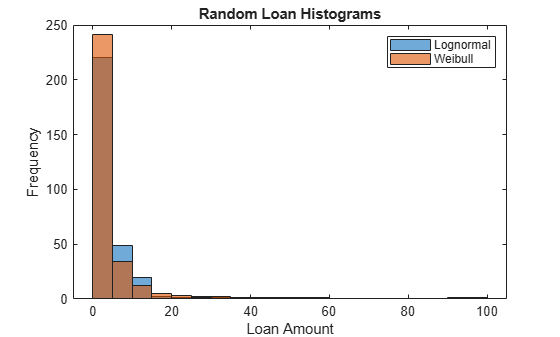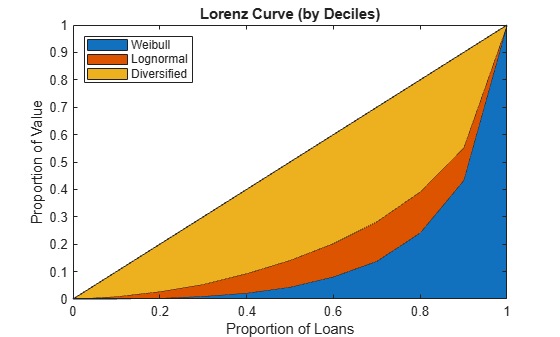Main Content

# Compare Concentration Indices for Random Portfolios

This example shows how to simulate random portfolios with different distributions and compare their concentration indices. For illustration purposes, a lognormal and Weibull distribution are used. The distribution parameters are chosen arbitrarily to get a similar range of values for both random portfolios.

Generate random portfolios with different distributions.

```rng('default'); % for reproducibility PLgn = lognrnd(1,1,1,300); PWbl = wblrnd(2,0.5,1,300);```

Display largest simulated loan value.

`fprintf('\nLargest loan Lognormal: %g\n',max(PLgn));`
```Largest loan Lognormal: 97.3582 ```
`fprintf('Largest loan Weibull: %g\n',max(PWbl));`
```Largest loan Weibull: 91.5866 ```

Plot the portfolio histograms.

```figure; histogram(PLgn,0:5:100) hold on histogram(PWbl,0:5:100) hold off title('Random Loan Histograms') xlabel('Loan Amount') ylabel('Frequency') legend('Lognormal','Weibull')```Compute and display the concentration measures.

```ciLgn = concentrationIndices(PLgn,'ID','Lognormal'); ciWbl = concentrationIndices(PWbl,'ID','Weibull'); disp([ciLgn;ciWbl])```
``` ID CR Deciles Gini HH HK HT TE ___________ ________ _____________ _______ ________ _________ _________ _______ "Lognormal" 0.066363 [1x11 double] 0.5686 0.013298 0.0045765 0.0077267 0.66735 "Weibull" 0.090152 [1x11 double] 0.72876 0.020197 0.0062594 0.012289 1.0944 ```
```ProportionLoans = 0:0.1:1; figure; area(ProportionLoans',[ciWbl.Deciles; ciLgn.Deciles-ciWbl.Deciles; ProportionLoans-ciLgn.Deciles]') axis([0 1 0 1]) legend('Weibull','Lognormal','Diversified','Location','NorthWest') title('Lorenz Curve (by Deciles)') xlabel('Proportion of Loans') ylabel('Proportion of Value')```## Support

#### Effective Model Risk Management with MATLAB

Download white paper